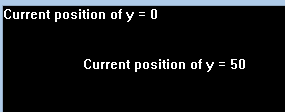# gety() function in C

• Difficulty Level : Medium
• Last Updated : 25 Jan, 2018

The header file graphics.h contains gety() function which returns the Y coordinate of the current position.
Syntax :

```int gety();
```

Example :

``````

Explanation : Initially, the Y coordinate of the current position is 0. On moving the coordinates using moveto() function, the Y coordinate changes to 50.

Below is the implementation of gety() function:

 `// C Implementation for gety()``#include ``#include `` ` `// driver code``int` `main()``{``    ``// gm is Graphics mode which is``    ``// a computer display mode that``    ``// generates image using pixels.``    ``// DETECT is a macro defined in``    ``// "graphics.h" header file``    ``int` `gd = DETECT, gm;``    ``char` `arr;`` ` `    ``// initgraph initializes the``    ``// graphics system by loading a``    ``// graphics driver from disk``    ``initgraph(&gd, &gm, ``""``);`` ` `    ``// sprintf stands for “String print”.``    ``// Instead of printing on console, it``    ``// store output on char buffer which``    ``// are specified in sprintf``    ``sprintf``(arr, ``"Current position of y = %d"``, ``                                     ``gety());`` ` `    ``// outtext function displays text at``    ``// current position.``    ``outtext(arr);`` ` `    ``// moveto function``    ``moveto(80, 50);``    ``sprintf``(arr, ``"Current position of y = %d"``,``                                     ``gety());`` ` `    ``// outtext function displays text at``    ``// current position.``    ``outtext(arr);`` ` `    ``getch();`` ` `    ``// closegraph function closes the``    ``// graphics mode and deallocates``    ``// all memory allocated by``    ``// graphics system .``    ``closegraph();`` ` `    ``return` `0;``}`

Output :

``````

My Personal Notes arrow_drop_up